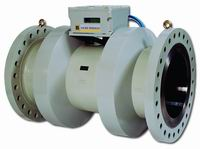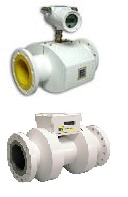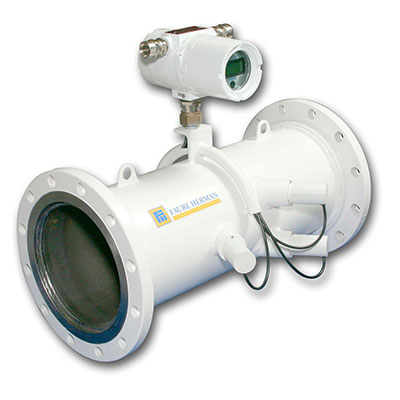Flow Control

# Flow Rate Measurement with an Ultrasonic Flowmeter

Giá bán: 0 đ

Lượt xem:Nhà Sản Xuất : Faure Herman

Flow Rate Measurement with an Ultrasonic Flowmeter

Measurement PrincipleThe measurement of the flow rate Q with an Ultrasonic Flowmeter (UFM) is the measurement of the velocity of fluid (VOF) multiplied by the section SThis measurement of the velocity of fluid (VOF) uses the transit times measurement of ultrasonic waves between two points and the difference of transit time, one point upstream and the other downstream. The first is one relative to the flow.

To go from upstream point A to downstream point B, the ultrasonic wave sent by transducer A and received by transducer B will take the time TAB that depends on:
• The Velocity of Sound (VOS) in the fluid
• The Velocity of Fluid (VOF)
• The angle θ from the fluid direction of the "beam" LAB
To go from downstream point B to upstream point A, the ultrasonic wave will take the time TBA.

We can deduct the velocity of fluid (VOF) (see formulas):Then the flowrate Q is proportional to the transit time difference ΔT and inversely proportional to the square of the average transit time T (see formulas):Flow Profile, Reynolds Number
The flow profile is the distribution of the velocity of fluid along the section.

The Reynolds Number, proposed in 1883 by Osborne Reynolds, characterizes the flow regime (laminar, transitional, turbulent).

The Reynolds Number represents the balance between inertial forces and viscous forces. It is defined as follows:VOF - Velocity of Fluid (m/s)
D - Internal Pipe Diameter (m)
v - Kinematic Viscosity of Fluid  v = ή/ρ (m2/s)
ρ - Fluid Density (kg/m3)
ή - Dynamic Viscosity of Fluid (Pa.s)

When the Reynolds Number is high (≥ 20,000), the flow type is turbulent (ex: water flow from tap)

When the Reynolds Number is low (≤ 2500), the flow type is laminar (ex: waste oil flow from box)

When the Reynolds Number is medium (2500 < Re < 20,000), the flow type is transitional (semi-turbulent, semi-laminar)

This drawing shows the Reynolds Number range for several products that the UFM can see for an 8" pipe size (DN200).When the Flow is laminar, the completely developed flow profile follows a logarithmic law, sharp and well developed in the middle of the flow profile. When the flow is turbulent, the completely developed flow profile follows a power law, not as developed and relatively flat in the middle of the flow profile.Calibration
The variation of the Reynolds Number from a change of flow rate or viscosity (variation of temperature, product, etc.) modifies the flow profile and then the "raw" measurement of the flow meter. Thanks to their multiple measurement beams, the UFM "watches" the flow profile shape, determine a Profile Factor (PF) and then is able to correct the measurement versus this flow profile. This correction curve can be predefined and fitted during the calibration, or completely determined during the calibration for maximum accuracy.

We can see that the correction only depends on the flow profile, so from the Reynolds Number.

Calibration in Reynolds

When the Reynolds Number is maximized, the flow profile is the flattest and the Profile Factor is minimized.

When the Reynolds Number increases, the flow profile develops, the Profile Factor increases and the measurement must have a calculated correction. This is the calibration in Reynolds, also known ascalibration in Profile Factor (PF).

The calibration in Reynolds allows for calibration at a different flow rate and product than the future used one, as long as it is at the same Reynolds Number, so it is the same flow profile and with the same measurement correction. When the product range on the field is wide, the selection of the flow rates and calibration productsis done in a way to span as close as possible the Reynolds Number range.

Example: UFM 8" (DN200), viscosity = 0.4cSt to 400cSt, flow rate = 220m3/h to 800m3h → Calibration with 4 products.Calibration in Velocity of Fluid VOF

The calibration in VOF is pertinent when the viscosity is stable. This is the case when the UFM sees only 1 product (e.g. gasoline from refinery). The Reynolds Number changes only with the velocity of fluid VOF, so with the flow rate.
Formulas
To go from upstream point A to downstream point B, the ultrasonic wave will take the time TAB:To go from downstream point B to upstream point A, the ultrasonic wave will take the time TBA:We can deduct the velocity of fluid VOF from the two previous equations:The flow rate Q is proportional to the transit time difference ΔT = TBA - TAB and inversely proportional to the square of the average transit time T = (TBA * TAB)1/2:## Custody Transfer - Ultrasonic Flowmeters

 Meters## Process Industry - Ultrasonic FlowmeterSản phẩm cùng loại

Giá bán: 0 VNĐ

Giá bán: 0 VNĐ

Giá bán: 0 VNĐ

Giá bán: 0 VNĐ

Giá bán: 0 VNĐ

Giá bán: 0 VNĐ

Giá bán: 0 VNĐ

Giá bán: 0 VNĐ

### Buttefly Valves_Wafer and lugged type (series L1 & L2) (sizes 16''- 48 '')

Giá bán: 0 VNĐ
Tin mới
Liên kết website
Thống kê truy cập^